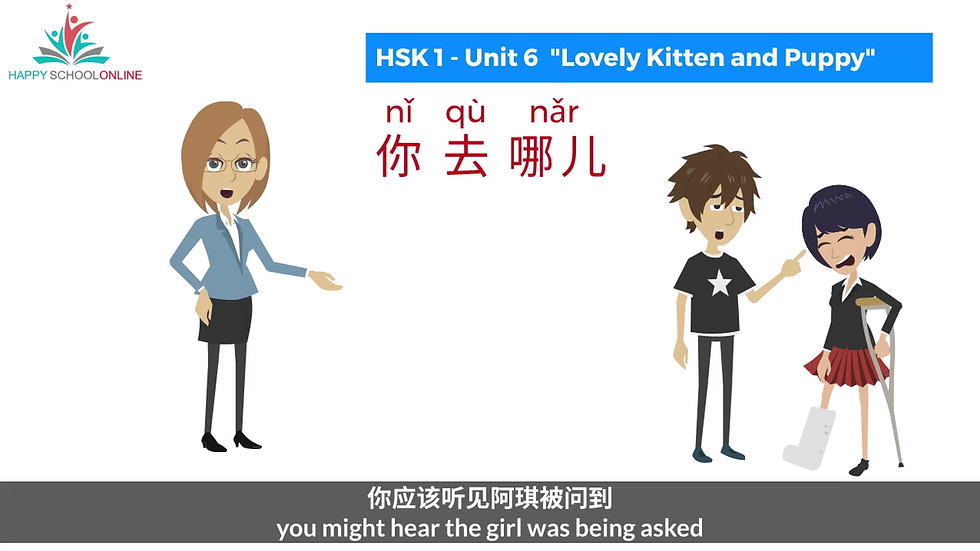## Where are You Going?

Learning Objectives

• Learn how to ask "Where are you going" in Mandarin.

### ​

In Mandarin, if you want to ask a person, “where are you going?”,  you could say “你去哪儿, nǐ qù nǎr”.  It is asking a place or location where the person will go in future.

• “你, nǐ” means “you”

• “去, qù” means “to go”.

• “哪儿, nǎr” is a question word, used to ask about a place or location where a person will go in future .

When putting these words together, "nǐ qù nǎr" is a common way to ask a person, “Where are you going?”

`The grammatical structure is: ``Subject + qù + qù?`` (Where does a person go?)`

In this structure, the Subject can be

• “我们, we”

• “他, he” or

• “他们, they”, etc.

For examples:

• 我们去哪儿?  (Where are we going?)

• 他去哪儿?      (Where is he going?)

• 他们去哪儿?  (Where are they going?)

If you want to ask a person, “where did you go?”,  you could say ”你去哪儿了, nǐ qù le nǎr?”. It is asking a place or location where the person already went in the past.

Do note that “了, le” is added after the verb “去, qù”. "le" is an auxiliary verb, placed after the verb, indicating that an action has already been completed.

`The grammatical structure is: ``Subject + 去 + 了+ 哪儿?`` (Where did a person go?)`

For examples:

• 你去了哪儿?     (Where did you go?)

• 他去了哪儿?     (Where did he go?)

• 他们去了哪儿?  (Where did they go?)